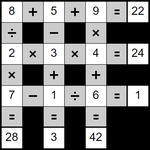Daily MathemaGridsToday's MathemaGrid PuzzlesPuzzle A - 0 - 1 - 2 - 3 random hints
Puzzle B - 0 - 1 - 2 - 3 random hints
Puzzle C - 0 - 1 - 2 - 3 random hints

All daily items change at midnight GMT.Notes• Objective / Rules
- Complete the grid using all of the digits 1 to 9.
- When completed all of the sums must be correct.
- The sums are solved strictly left to right, up to down.
- The normal order of mathematical operations is ignored.
- For example 2 + 5 x 9 is calculated as (2 + 5) x 9 = 63.
- There are no ÷ 1 in the puzzle.
- There are no x 1 in the puzzle (although there might be 1 x).
- At no point will any calculation go below zero, or fractional.

• Help
Read the help/walkthrough page on MathemaGrids for a more detailed explanation and a walkthrough.

• Pencil Marks
What is a pencil mark? Pencil marks can help you keep track of your own thinking, simply enter more than one digit/letter into each square, just like you might do if you were using pen and paper. The size of the digits/letters is smaller when there is more than one digit/letter in a square. A square might contain more pencil mark digits/letters than can be shown.

• Checking
If you click 'Check' the system will check for incorrect squares. If 'Show mistakes when checking' is checked they will be marked in red.

• Uniqueness
Each puzzle has exactly one solution. If you think you've found another solution, then please double check the rules.

• JavaScript